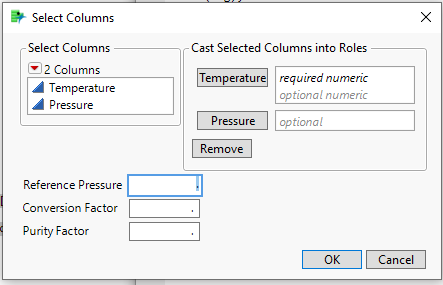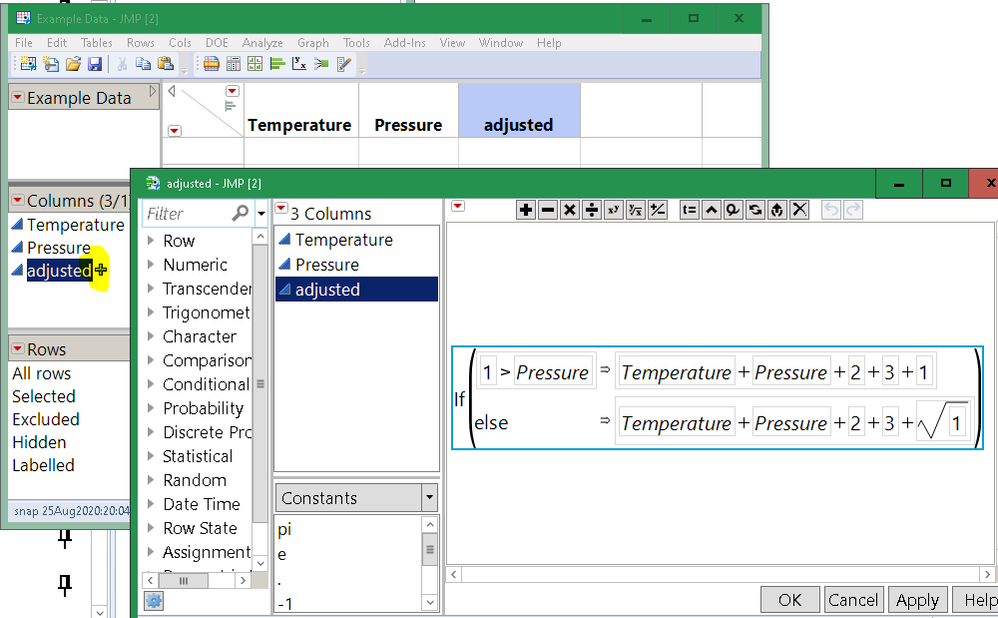Choose Language Hide Translation Bar
Highlighted

## Need Help with JSL for Pressure Compensated Temperature Formula

Hello,

I am looking for an example or some guidance on how to get started writing JSL for the following Pressure Compensated Temperature:

• Create a UI to select a Temperature column and Pressure Column.  Also have user input a Reference Pressure, Conversion Factor, Purity factor.
• Calculate the Pressure Compensated Temperature for each row using several IF statements and mathematical expressions using the UI data.
• Create a new column and fill it in with the Pressure Compensated Temperature calculated above.

Any help is appreciated!

2 ACCEPTED SOLUTIONS

Accepted Solutions
Highlighted

## Re: Need Help with JSL for Pressure Compensated Temperature Formula

2. I can help you a bit with the UI, but "Calculate the Pressure Compensated Temperature for each row using several IF statements and mathematical expressions using the UI data."  is too vague to really guide you.Here is the JSL for the above.  You will just need to take the info from the window and plug it into your formulas.  Read the sections in the documentation on Column Dialog() and on New Column()

``````names default to here(1);
dtList = New Table("Example Data",
new column("Temperature"),
new column("Pressure")
);

dlg = Column Dialog(
temperatureCol = ColList( "Temperature", Min Col( 1 ), Max Col( 2 ), Data Type( "Numeric" ) ),
pressureCol = ColList( "Pressure", Max Col( 1 ), Modeling Type( {"Continuous", "Multiple Response"} ) ),
Line Up( 2,
Text Box( "Reference Pressure" ), refPressure = EditNumber( . ),
Text Box( "Conversion Factor" ), conFactor = EditNumber( . ),
Text Box( "Purity Factor" ), purFactor = EditNumber( . )
));

show(dlg);``````
Jim
Highlighted

## Re: Need Help with JSL for Pressure Compensated Temperature Formula

Cool! not sure I ever used column dialog, but it seems perfect for this job.

After you get Jim's example running, try some code like this to add a formula column to your table.

``````Eval( // run the code below
Eval Expr( // convert the Expr(...) below into a hard coded value
// eval(evalexpr(expr(...))) is a pattern for getting values from the dlg INTO
// A FORMULA. if you follow this pattern, the formula will be self contained.
formula( // random formula that uses all the variables AND shows an if() stmt
If( Expr( dlg["refPressure"] ) > Expr( dlg["pressureCol"] ),
Expr( dlg["temperatureCol"] ) + // first item of list
Expr( dlg["pressureCol"] ) + //
Expr( dlg["conFactor"] ) + // simple value
Expr( dlg["purFactor"] ) + //
Expr( dlg["refPressure"] )// do the ususal stuff...
, // else refPressure <= pressureCol...
Expr( dlg["temperatureCol"] ) + // first item of list
Expr( dlg["pressureCol"] ) + //
Expr( dlg["conFactor"] ) + // simple value
Expr( dlg["purFactor"] ) + //
Sqrt( Expr( dlg["refPressure"] ) )// do something extra for low ref
)
)
)
)
);``````

There are several things going on here.

• formula() does not evaluate its argument, so you have to give it some help
• I fall back on the eval(evalexpr(expr(...))) technique. There are other ways.
• you said you need an if(...) so I made one. You can use any JSL you like that produces a simple number for a result. The result of an if() is the last value it computes.
• if you need to use a variable more than once in your formula, look at the formula above.
• Clearly my formula is not the one you are looking for!
• For simple formulas, you don't need all the evalexpr stuff. formula(:pressure * :temperature) works fine. But in this example, you don't know the names of the columns; instead you know the name of a variable that holds the name of the column.

for reference, the dlg returns this list:

``````// this is what the dlg returns; the columns are a list of 1 item
// and the parameters are simple values:
//
// {temperatureCol = {:Temperature}, pressureCol = {:Pressure},
// Text Box, refPressure = 3, Text Box, conFactor = 33,
// Text Box, purFactor = 333, Button( 1 )}``````

Finally, be sure to look at the formula you created using the formula editor to make sure it is really what you expect!Click the + next to the column name to open the formula in the editor

Craige
4 REPLIES 4
Highlighted

## Re: Need Help with JSL for Pressure Compensated Temperature Formula

2. I can help you a bit with the UI, but "Calculate the Pressure Compensated Temperature for each row using several IF statements and mathematical expressions using the UI data."  is too vague to really guide you.Here is the JSL for the above.  You will just need to take the info from the window and plug it into your formulas.  Read the sections in the documentation on Column Dialog() and on New Column()

``````names default to here(1);
dtList = New Table("Example Data",
new column("Temperature"),
new column("Pressure")
);

dlg = Column Dialog(
temperatureCol = ColList( "Temperature", Min Col( 1 ), Max Col( 2 ), Data Type( "Numeric" ) ),
pressureCol = ColList( "Pressure", Max Col( 1 ), Modeling Type( {"Continuous", "Multiple Response"} ) ),
Line Up( 2,
Text Box( "Reference Pressure" ), refPressure = EditNumber( . ),
Text Box( "Conversion Factor" ), conFactor = EditNumber( . ),
Text Box( "Purity Factor" ), purFactor = EditNumber( . )
));

show(dlg);``````
Jim
Highlighted

## Re: Need Help with JSL for Pressure Compensated Temperature Formula

Cool! not sure I ever used column dialog, but it seems perfect for this job.

After you get Jim's example running, try some code like this to add a formula column to your table.

``````Eval( // run the code below
Eval Expr( // convert the Expr(...) below into a hard coded value
// eval(evalexpr(expr(...))) is a pattern for getting values from the dlg INTO
// A FORMULA. if you follow this pattern, the formula will be self contained.
formula( // random formula that uses all the variables AND shows an if() stmt
If( Expr( dlg["refPressure"] ) > Expr( dlg["pressureCol"] ),
Expr( dlg["temperatureCol"] ) + // first item of list
Expr( dlg["pressureCol"] ) + //
Expr( dlg["conFactor"] ) + // simple value
Expr( dlg["purFactor"] ) + //
Expr( dlg["refPressure"] )// do the ususal stuff...
, // else refPressure <= pressureCol...
Expr( dlg["temperatureCol"] ) + // first item of list
Expr( dlg["pressureCol"] ) + //
Expr( dlg["conFactor"] ) + // simple value
Expr( dlg["purFactor"] ) + //
Sqrt( Expr( dlg["refPressure"] ) )// do something extra for low ref
)
)
)
)
);``````

There are several things going on here.

• formula() does not evaluate its argument, so you have to give it some help
• I fall back on the eval(evalexpr(expr(...))) technique. There are other ways.
• you said you need an if(...) so I made one. You can use any JSL you like that produces a simple number for a result. The result of an if() is the last value it computes.
• if you need to use a variable more than once in your formula, look at the formula above.
• Clearly my formula is not the one you are looking for!
• For simple formulas, you don't need all the evalexpr stuff. formula(:pressure * :temperature) works fine. But in this example, you don't know the names of the columns; instead you know the name of a variable that holds the name of the column.

for reference, the dlg returns this list:

``````// this is what the dlg returns; the columns are a list of 1 item
// and the parameters are simple values:
//
// {temperatureCol = {:Temperature}, pressureCol = {:Pressure},
// Text Box, refPressure = 3, Text Box, conFactor = 33,
// Text Box, purFactor = 333, Button( 1 )}``````

Finally, be sure to look at the formula you created using the formula editor to make sure it is really what you expect!Click the + next to the column name to open the formula in the editor

Craige
Highlighted

## Re: Need Help with JSL for Pressure Compensated Temperature Formula

Hi,

What do you mean by "Pressure Compensated Temperature"? More specific details would be easier for support

Highlighted

## Re: Need Help with JSL for Pressure Compensated Temperature Formula

Thank you both very much!  This is exactly what I needed.  This may not be optimal, but I ended up creating intermediate columns with formulas to derive the pressure compensated temperature (pct).  I didn't want several intermediate columns in my data table, so I removed the formula from the pct and deleted the intermediate columns.  If I ever add more rows of data I'll have to rerun the script.

I pasted the code I came up with below (but note that I had to change all the constants / coefficients since it's for my work)

``````//Script for creating a pressure compensated temperature tag
//Rev 1 8/25/20

names default to here(1);
/*dtList = New Table("Example Data",
new column("Temperature"),
new column("Pressure")
);*/
dtList = CurrentDataTable();

dlg = Column Dialog(
temperatureCol = ColList( "Temperature", Max Col( 1 ), Modeling Type( {"Continuous", "Multiple Response"} ) ),
pressureCol = ColList( "Pressure", Max Col( 1 ), Modeling Type( {"Continuous", "Multiple Response"} ) ),
Line Up( 2,
Text Box( "Reference Pressure" ), refPressure = EditNumber( . ),
Text Box( "Conversion Factor" ), conFactor = EditNumber( 1 ),
Text Box( "Purity Factor" ), purFactor = EditNumber( 1 ),
Text Box( "Pressure Units"), unitsP = comboBox( {"Gauge","Absolute"}),
Text Box( "PComp Tag Name"), pcompTag = EditText( "PComp Tag" )
),
Text Box("
**Reference Pressure is the base pressure and must be in the same units as the pressure tag.

**Pressure Units is a logical to indicate if the pressure being measured is absolute or gauge.  For most
situations, the pressure will be measure in terms of gauge.  However, vacuum pipestills typically use absolute.

**PComp Tag Name will be the column name of the new Pressure Compensate Temperature tag.
"),
);

show(dlg);

//Absolute needs to be 0 and Gauge needs to be 1.  The combo box has Absolute as 2 and Gauge as 1,
If( dlg["unitsP"] == 1, dlg["unitsP"] = 1, dlg["unitsP"] = 0 );
//Write("unitsP equals: ", dlg["unitsP"]);

Eval( // run the code below
Eval Expr( // convert the Expr(...) below into a hard coded value
// eval(evalexpr(expr(...))) is a pattern for getting values from the dlg INTO
// A FORMULA. if you follow this pattern, the formula will be self contained.
dtList << New Column( "PA",
formula( // random formula that uses all the variables AND shows an if() stmt
Expr( dlg["purFactor"] ) *
(Expr( dlg["unitsP"] ) + Expr( dlg["pressureCol"] ) * Expr( dlg["purFactor"] ) / 12)
)
)
)
);
Eval( // run the code below
Eval Expr(
dtList << New Column( "PB",
formula( // random formula that uses all the variables AND shows an if() stmt
Expr( dlg["refPressure"] ) * Expr( dlg["conFactor"] ) / 12 + Expr( dlg["unitsP"] )
)
)
)
);

Eval(
Eval Expr(
dtList << New Column( "TA",
formula( // random formula that uses all the variables AND shows an if() stmt
Expr( dlg["temperatureCol"] ) + 400
)
)
)
);
New Column( "AA",
formula(
If( :PA >= 1.1111 ,
(4 - LOG10(:PA)) / (2000 - 25*LOG10(:PA)) - 0.0001
, // else
(3- LOG10(:PA)) / (2000 - 25*LOG10(:PA)) - 0.0001
)
)
);
New Column( "AB",
formula(
If( :PB >= 1.1111 ,
(4 - LOG10(:PB)) / (2000 - 25*LOG10(:PB)) - 0.0001
, // else
(3 - LOG10(:PB)) / (2000 - 100*LOG10(:PB)) - 0.0001
)
)
);
New Column( "DEN",
formula(
:AB / :AA * ((1.1 / :TA) - 0.0001) + 0.0001
)
);
New Column( "TB",
formula(
1.1 / :DEN - 400
)
);

Column("TB") << delete formula;
Column("TB") << Set Name( dlg["pcompTag"] );

dtList << delete columns( {"PA","PB","TA","AA","AB","DEN"} );``````
Article Labels